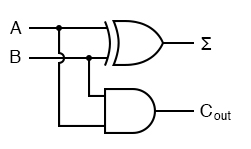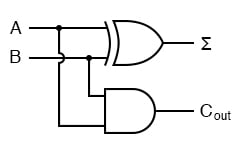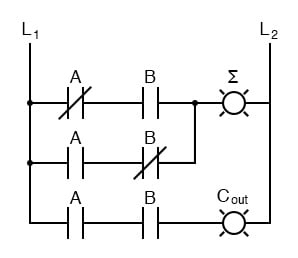## What is the half-adder useful for?

Adding more than one binary digit quantities.

## Chapter 9 - Combinational Logic Functions

As a first example of useful combinational logic, let’s build a device that can add two binary digits together. We can quickly calculate what the answers should be:

0 + 0 = 0          0 + 1 = 1          1 + 0 = 1          1 + 1 = 102

So we will need two inputs (a and b) and two outputs. The low order output will be called Σ because it represents the sum, and the high order output will be called Cout because it represents the carry out. The truth table is:

 A B Σ Cout 0 0 0 0 0 1 1 0 1 0 1 0 1 1 0 1

Simplifying boolean equations or making some Karnaugh map will produce the same circuit shown below, but start by looking at the results. The Σ column is our familiar XOR gate, while the Cout column is the AND gate. This device is called a half-adder for reasons that will make sense in the next section.